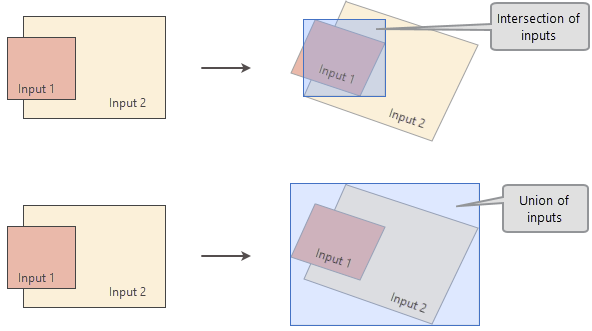# How the analysis window is determined in Spatial Analyst

The analysis window is the geographic area where the analytical operation is performed on the data. During raster analysis, only the cells that fall within the analysis window are processed to create the output. The analysis window depends on several things, including the following:

• Output spatial reference
• Initial analysis extent derived from input dataset and extent environment, projected to output spatial reference
• Output cell size and snap raster specified in the environment

If a dataset is specified in the Mask environment, it does not influence the determination of the analysis window.

Before raster analysis is performed, the analysis window is determined by multiple steps in the following order:

• Determine the output spatial reference.
• Determine the initial analysis extent.
• Determine the output cell size.
• Determine the final analysis window combining initial analysis extent, output cell size, and snap raster if specified.

## How the output spatial reference is determined

The output spatial reference will be the same as the Output Coordinate System environment, if it has been set. If the output coordinate system hasn't been specified, the output spatial reference will be determined using the following rules:

• If the tool has one or multiple rasters, with or without feature inputs, the spatial reference of the first raster data will be used.
• If the tool only has feature input, the spatial reference of the features will be used.
• If the tool has no input, such as Create Constant Raster, the spatial reference of the map will be used, if it exists. Otherwise, it will be Unknown.

## How the initial analysis extent is determined

The initial extent is determined in the output coordinate system by projecting all datasets used in the analysis to the same coordinate system. It can be defined by numeric values or obtained from an existing raster dataset.

If the extent hasn’t been explicitly specified in the tool parameter, it is derived from the Extent environment if it has been set. Otherwise, the initial extent is derived from the inputs, which could be the intersection of or union of input dataset extents, based on the tool. See the figure below for an illustration of these two scenarios.Initial analysis extent as derived from the intersection and union of inputs.

For some tools, such as Zonal Statistics, the intersected extent is further adjusted to align cells with one of the input rasters.

## How the output cell size is determined

The output cell size is determined in the output coordinate system by projecting all datasets used in the analysis, and based on the Cell Size Projection Method selected. It can also be defined by a numeric value or obtained from an existing raster dataset. If the cell size is specified using a numeric value, the value is assumed to be in the units of the output spatial reference.

If the cell size hasn't been explicitly specified as the parameter value, the Cell Size environment will be used if specified. Otherwise, it will be determined using the following rules:

• If the tool has one raster input, with or without feature inputs, the cell size of the raster dataset is used.
• If the tool has multiple rasters, with or without feature inputs, the output cell size will be the largest cell size of the input rasters.
• If the tool only has a feature input and the Snap Raster environment has been set, the cell size of the snap raster is used. If no snap raster is set, the cell size is calculated from the shorter of the width or height of the extent divided by 250, where the extent is in the output coordinate system specified in the environment.
• If the tool has no input data, such as Create Constant Raster, and the snap raster environment has been set, the cell size of the snap raster is used. If no snap raster is set, the cell size is 1.

## How the final analysis extent is determined

The final analysis window is determined by combining the initial analysis extent, the output cell size, and the snap raster. When no snap raster is specified, the lower left corner of the initial analysis extent will automatically become the lower left corner of the final analysis window. If a snap raster environment is specified, the lower left corner of the final analysis window is moved to the nearest snap raster cell corner in such a way that the new lower left corner is outside of the initial analysis extent, as shown below: